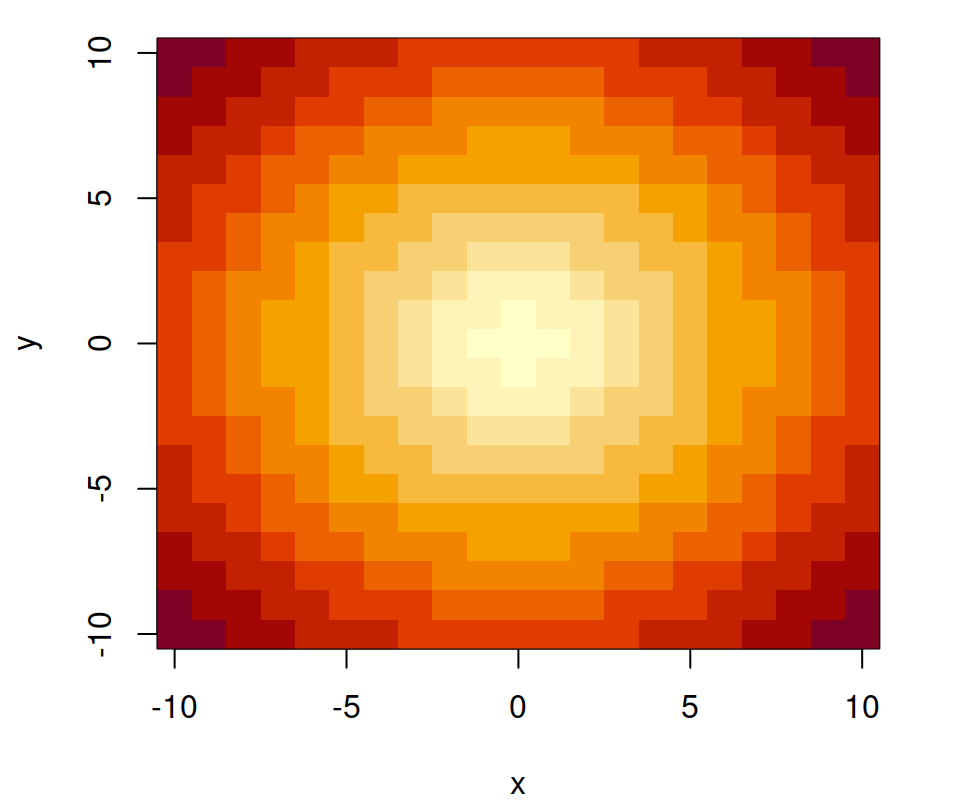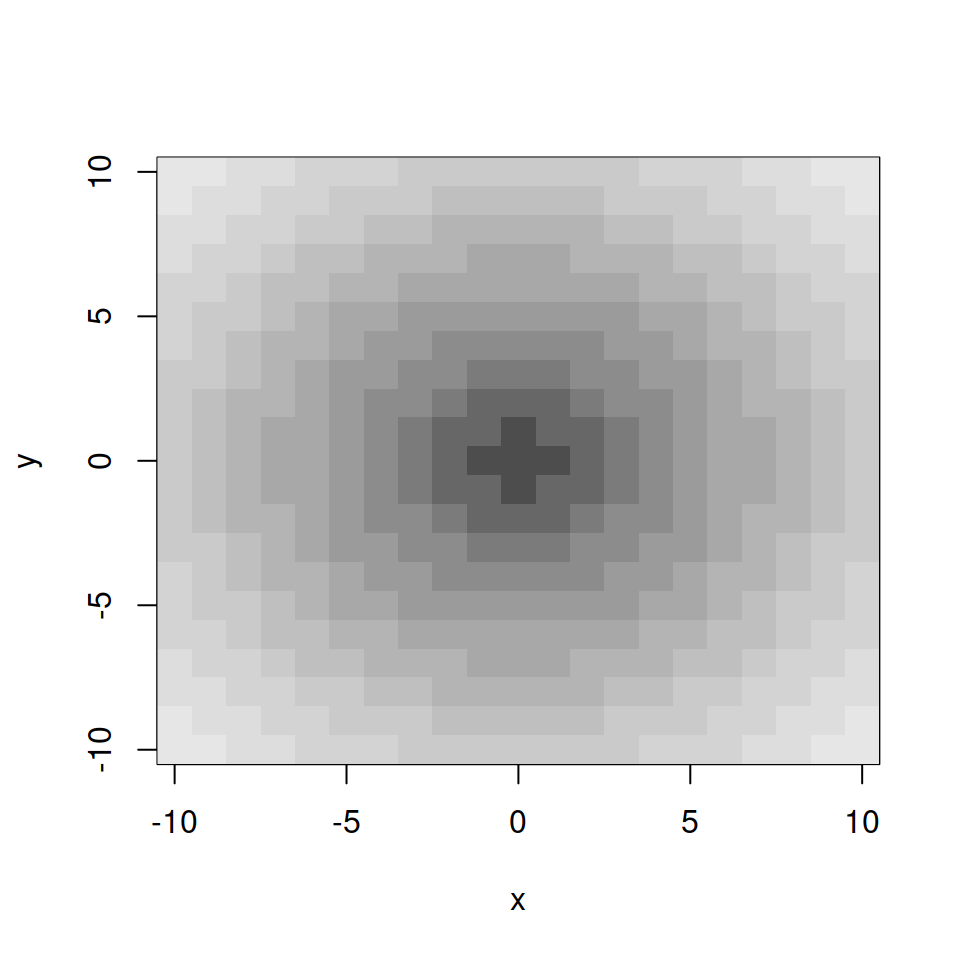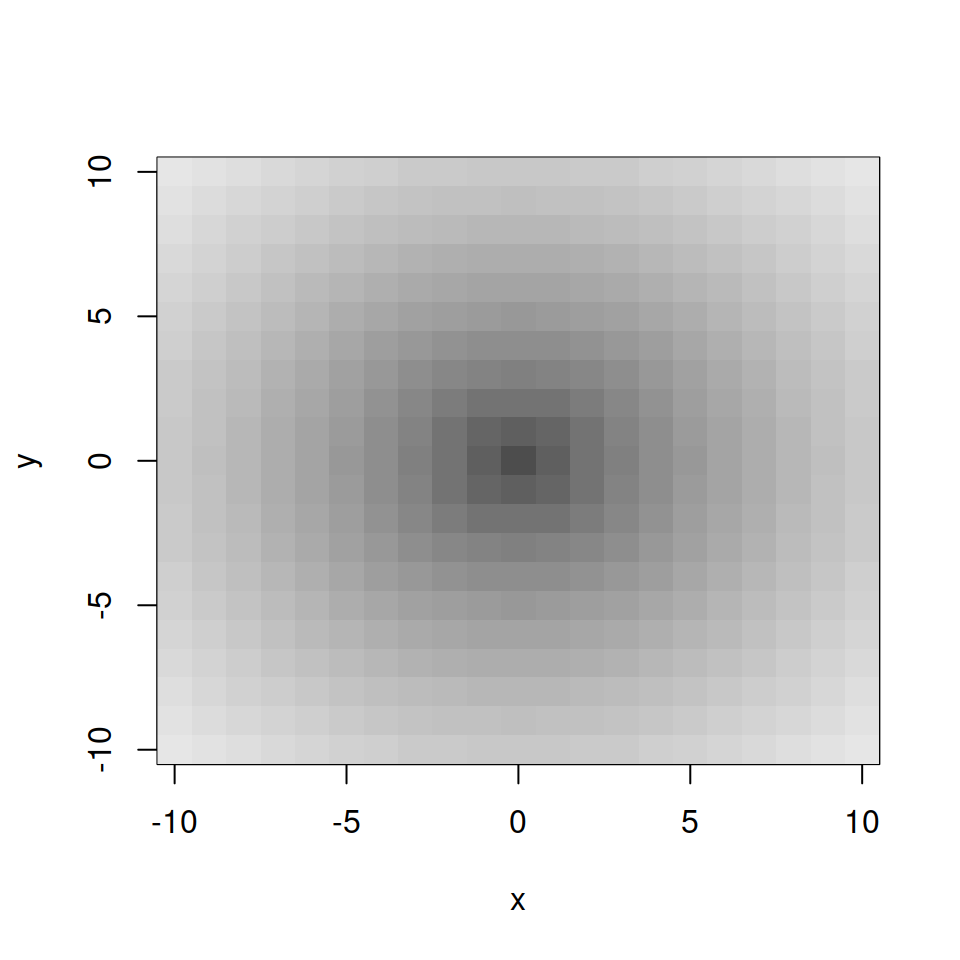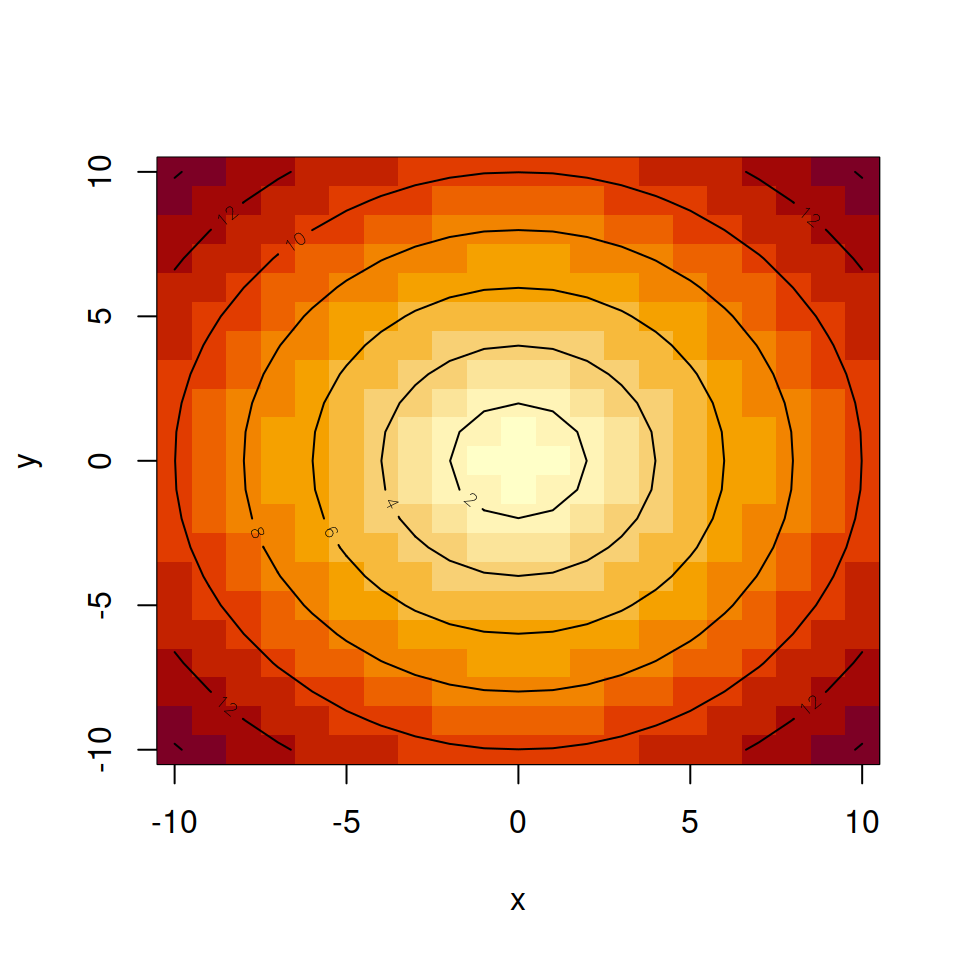# image function in R

## Basic color image

The `image` function can be used to display spatial data (images), creating a grid of colored rectangles based on the values of the matrix `z`. The arguments `x` and `y` can be used to specify the grid where the values of `z` are measured.

``````# Data
x <- -10:10
y <- -10:10
z <- sqrt(outer(x ^ 2, y ^ 2, "+"))

image(x, y, z)

# You can also type, the following
# but the axes will be between 0 and 1
image(z)``````## Color customizationThe `col` argument allows customizing the color palette of the image. You can pass as variable a function such as `gray.colors`, `topo.colors`, `hcl.colors` or a similar function. The default value is `hcl.colors(12, "YlOrRd", rev = TRUE)`.

``````# Data
x <- -10:10
y <- -10:10
z <- sqrt(outer(x ^ 2, y ^ 2, "+"))

image(x, y, z, col = gray.colors(12))``````Note that if you increase the number of values the color image will be smoothed.

``````# Data
x <- -10:10
y <- -10:10
z <- sqrt(outer(x ^ 2, y ^ 2, "+"))

image(x, y, z, col = gray.colors(50))``````

## Overlaying a contour

It is possible to overlay the contour lines over the color image, passing the same data to the function and specifying `add = TRUE`.

``````# Data
x <- -10:10
y <- -10:10
z <- sqrt(outer(x ^ 2, y ^ 2, "+"))

image(x, y, z)
contour(x, y, z, add = TRUE)``````